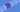# JavaScript - Variables

### 2020-05-16

Variables allow programmers to store information to use later in the program. For example, you may want to store the a number in a variable called total, then as you are working through a list of items in a shopping car, you would want to increment that number. Let's take a quick look at how to use variables in Javascript.

## Const Variables

``````const age = 27
const name = "Keith"
const salary = 1000.00

console.log(age) # prints 27``````

The first way to declare variables is using the `const` keyword followed by the name of the variable, the `=` assignment operation and then the value.

From our example, we assigned the number 27 to a variable called age. We can then use that variable later as showing in the console.log statement.

Const will prevent code in the future from changing the value. You should try to use constants when you can as they are "safer." The reason for such things will be discussed in later lessons.

## Let Variables

``````const cost = 100;

let total = 10;

total = total + cost;

console.log(total); // prints 110``````

Now, the next way to declare variables is with the `let` keyword. When using the let keyword, you are allowed to change your variables later. As in the example, we have a variable name total, and we increase it's value by adding the cost.

And that's it! You can try this lesson for yourself here: https://jsfiddle.net/theholliday/gkLyh1rf/

GoTea - KoalaTea